Technical Article

# The Nyquist–Shannon Theorem: Understanding Sampled Systems

May 06, 2020 by Robert Keim

## The Nyquist sampling theorem, or more accurately the Nyquist-Shannon theorem, is a fundamental theoretical principle that governs the design of mixed-signal electronic systems.

Modern technology as we know it would not exist without analog-to-digital conversion and digital-to-analog conversion. In fact, these operations have become so commonplace that it sounds like a truism to say that an analog signal can be converted to digital and back to analog without any significant loss of information.

But how do we know that this is indeed the case? Why is sampling a non-destructive operation, when it appears to discard so much signal behavior that we observe between the individual samples?

How on earth can we start with a signal that looks like this: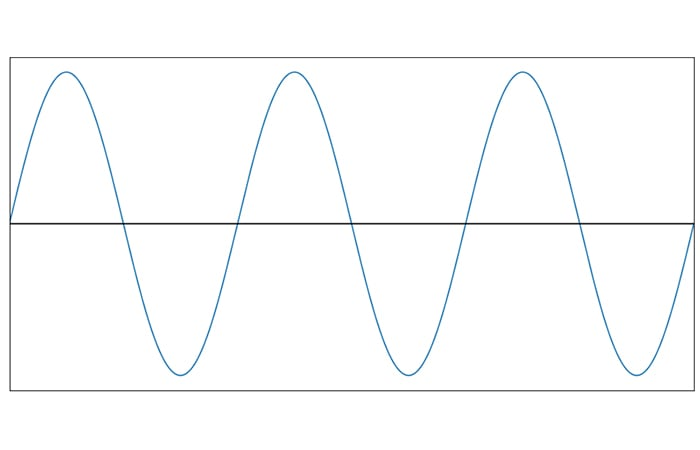And digitize it into this: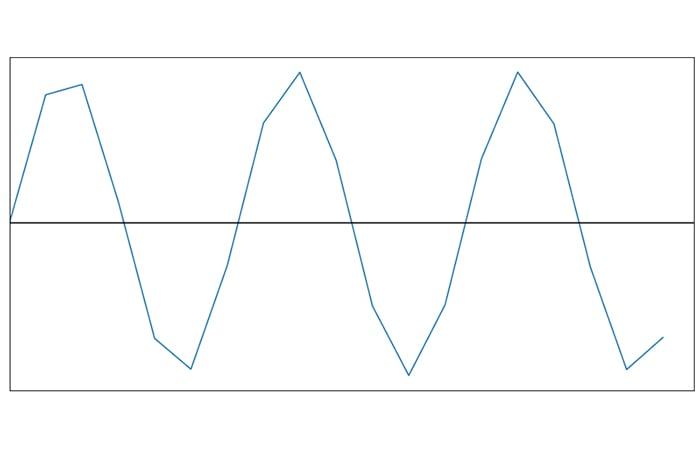And then dare to claim that the original signal can be restored with no loss of information?

### The Nyquist–Shannon Theorem

Such a claim is possible because it is consistent with one of the most important principles of modern electrical engineering:

If a system uniformly samples an analog signal at a rate that exceeds the signal’s highest frequency by at least a factor of two, the original analog signal can be perfectly recovered from the discrete values produced by sampling.

There is much more that needs to be said about this theorem, but first, let’s try to figure out what to call it.

### Shannon? Nyquist? Kotelnikov? Whittaker?

I am certainly not the person to decide who deserves the most credit for formulating, demonstrating, or explaining the Shannon–Nyquist–Kotelnikov–Whittaker Theory of Sampling and Interpolation. All four of these individuals had some sort of prominent involvement.

However, it does appear that the role of Harry Nyquist has been extended beyond its original significance. For example, in Digital Signal Processing: Fundamentals and Applications by Tan and Jiang, the principle stated above is identified as the “Shannon sampling theorem,” and in Microelectronic Circuits by Sedra and Smith, I find the following sentence: “The fact that we can do our processing on a limited number of samples … while ignoring the analog-signal details between samples is based on … Shannon’s sampling theorem.”

Thus, we probably should avoid using “the Nyquist sampling theorem” or “Nyquist’s sampling theory.” If we need to associate a name with this concept, I suggest that we include only Shannon or both Nyquist and Shannon. And in fact, maybe it’s time to transition to something more anonymous, such as “Fundamental Sampling Theorem.”

If you find this somewhat disorienting, remember that the sampling theorem stated above is distinct from the Nyquist rate, which will be explained later in the article. I don’t think that anyone is trying to separate Nyquist from his rate, so we end up with a good compromise: Shannon gets the theorem, and Nyquist gets the rate.

### Sampling Theory in the Time Domain

If we apply the sampling theorem to a sinusoid of frequency fSIGNAL, we must sample the waveform at fSAMPLE ≥ 2fSIGNAL if we want to enable perfect reconstruction. Another way to say this is that we need at least two samples per sinusoid cycle. Let’s first try to understand this requirement by thinking in the time domain.

In the following plot, the sinusoid is sampled at a frequency that is much higher than the signal frequency.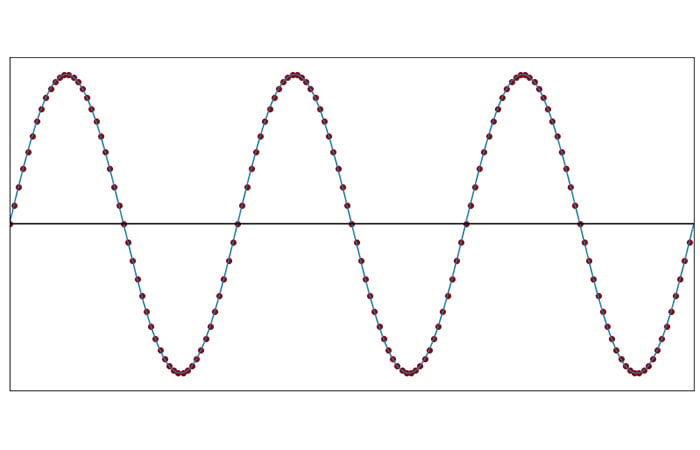##### Each circle represents a sampling instant, i.e., a precise moment at which the analog voltage is measured and converted into a number.

To better visualize what this sampling procedure has given us, we can plot the sample values and then connect them with straight lines. The straight-line approximation shown in the next plot looks exactly like the original signal: the sampling frequency is very high relative to the signal frequency, and consequently the line segments are not noticeably different from the corresponding curved sinusoid segments.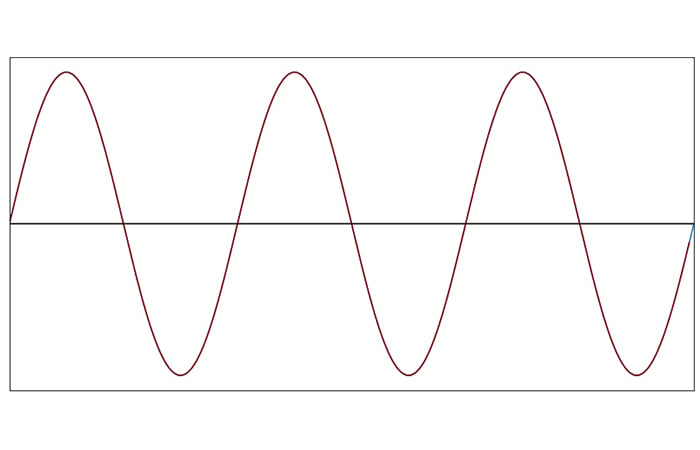As we reduce the sampling frequency, the appearance of the straight-line approximation diverges from the original.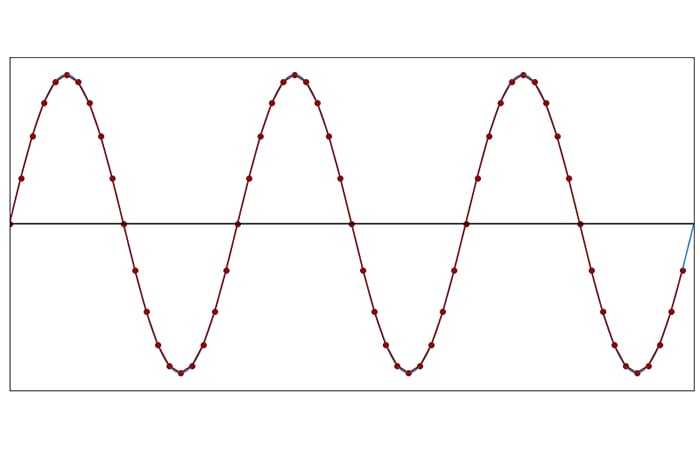##### 20 samples per cycle (fSAMPLE = 20fSIGNAL)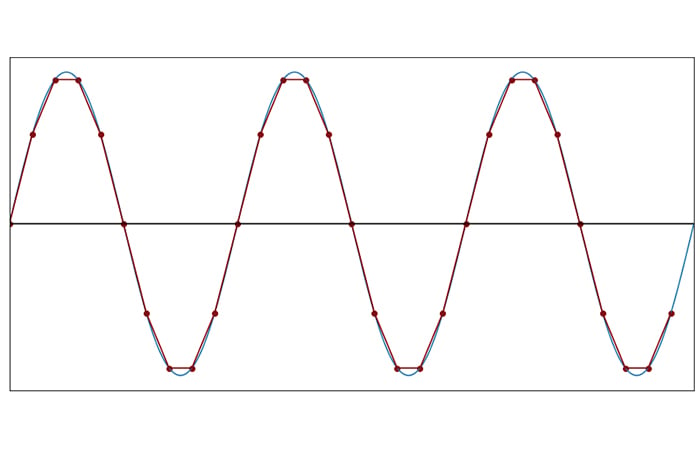##### 10 samples per cycle (fSAMPLE = 10fSIGNAL)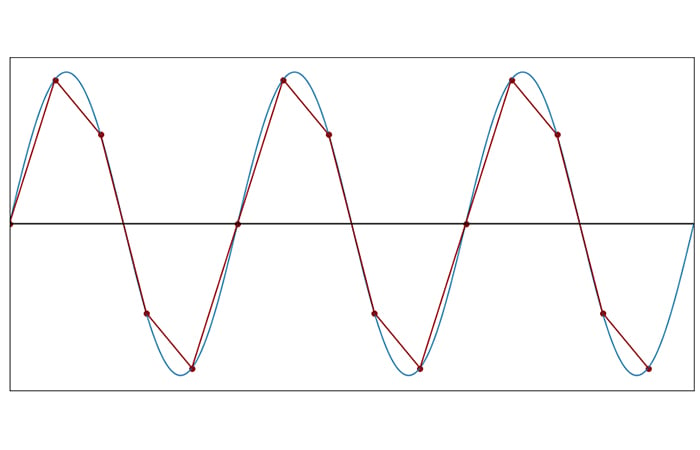##### 5 samples per cycle (fSAMPLE = 5fSIGNAL)

At fSAMPLE = 5fSIGNAL, the discrete-time waveform is no longer a pleasing representation of the continuous-time waveform. However, notice that we can still clearly identify the frequency of the discrete-time waveform. The cyclic nature of the signal has not been lost.

#### The Threshold: Two Samples per Cycle

The data points produced by sampling will continue to retain the cyclic nature of the analog signal as we decrease the number of samples per cycle below five. However, eventually we reach a point at which frequency information is corrupted. Consider the following plot: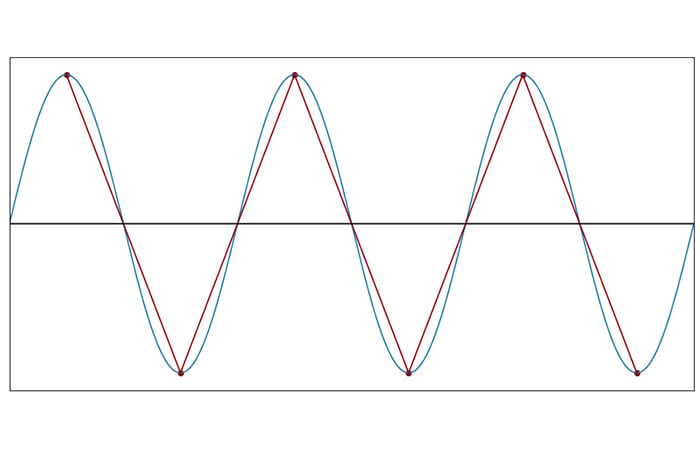##### 2 samples per cycle (fSAMPLE = 2fSIGNAL)

With fSAMPLE = 2fSIGNAL, the sinusoidal shape is completely gone. Nevertheless, the triangle wave created by the sampled data points has not altered the fundamental cyclical nature of the sinusoid. The frequency of the triangle wave is identical to the frequency of the original signal.

However, as soon as we reduce the sampling frequency to the point at which there are fewer than two samples per cycle, this statement can no longer be made. Two samples per cycle, for the highest frequency in the original waveform, is therefore a critically important threshold in mixed-signal systems, and the corresponding sampling frequency is called the Nyquist rate:

If we sample an analog signal at a frequency that is lower than the Nyquist rate, we will not be able to perfectly reconstruct the original signal.

The next two plots demonstrate the loss of cyclical equivalency that occurs when the sampling frequency drops below the Nyquist rate.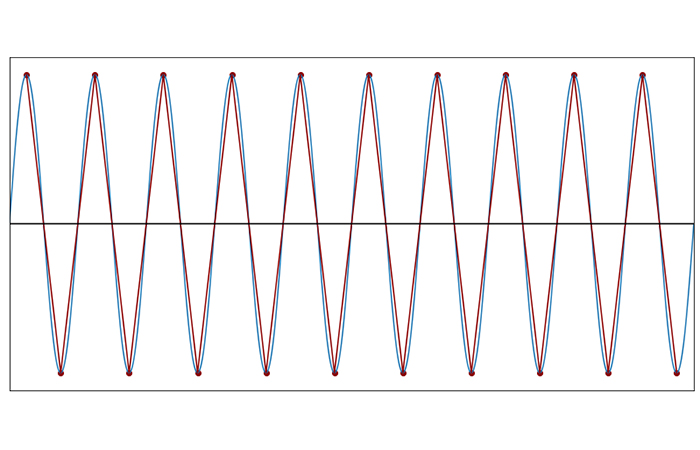##### 2 samples per cycle (fSAMPLE = 2fSIGNAL)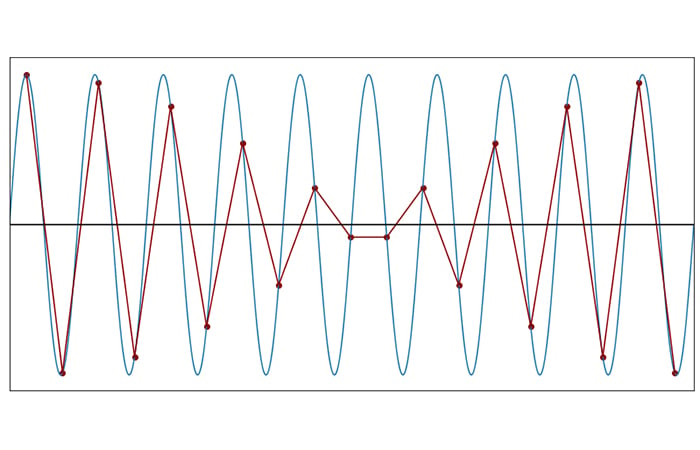##### 1.9 samples per cycle (fSAMPLE = 1.9fSIGNAL)

At fSAMPLE = 1.9fSIGNAL, the discrete-time waveform has acquired fundamentally new cyclical behavior. Full repetition of the sampled pattern requires more than one sinusoid cycle.

However, the effect of insufficient sampling frequency is somewhat difficult to interpret when we have 1.9 samples per cycle. The next plot makes the situation more clear.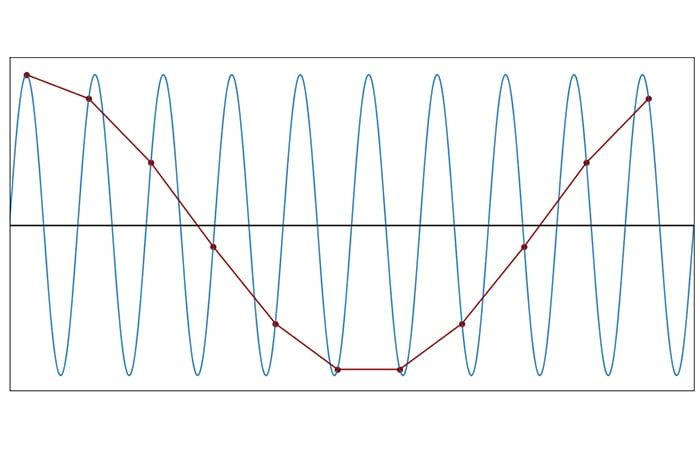##### 1.1 samples per cycle (fSAMPLE = 1.1fSIGNAL)

If you knew nothing about a sinusoid and performed an analysis using the discrete-time waveform resulting from sampling at 1.1fSIGNAL, you would form seriously erroneous ideas about the frequency of the original signal. Furthermore, if all you have is the discrete data, it is impossible to know that frequency characteristics have been corrupted. Sampling has created a new frequency that was not present in the original signal, but you don’t know that this frequency was not present.

The bottom line is this: When we sample at frequencies below the Nyquist rate, information is permanently lost, and the original signal cannot be perfectly reconstructed.

### Conclusion

We’ve covered the Shannon sampling theorem and the Nyquist rate, and we tried to gain some insight into these concepts by looking at the effect of sampling in the time domain. In the next article, we’ll explore this topic from the perspective of the frequency domain.• R
RjvBmn May 08, 2020

Nyquist theorem wonderfully explained in time-domain !

Like.
• S
swr999 May 08, 2020

I had always thought you need to satisfy the theorem by sampling “more than” twice the frequency of the highest frequency content in the signal you’re sampling?

Like.
•brianrho May 08, 2020

Nice. But if it’s called the Nyquist rate, then surely the other must also be called the Nyquist Theorem, since the rate fs >= 2f_signal is practically a mathematical representation of the theorem.

Like.
• M
mugwort May 09, 2020

This is somewhat inaccurate as sampling the highest frequency with only 2 samples only works if you take those samples at the peaks of the wave, if you take the samples at the nodes the wave becomes 0.. for this reason if you sampled the frequency at say 2.1x sampling rate it would also oscillate in amplitude the same way 1.9x does, the reason there is no loss in amplitude for the top octave in real music (with many superimposed frequencies) remains a mystery to me, but it cannot be explained with this theorem since it does not hold for single sine waves, you can test this by sampling a single 19khz sine wave at 44.1kbps and seeing that the amplitude does actually oscillate as it cycles in and out of phase, despite being over 2x the frequency.

Like.
• B
Bernie Hutchins May 09, 2020
SWR999, You are quite correct - it has to be GREATER THAN twice the highest frequency else the amplitude is arbitrary, and could even be zero if samples fall at the zero-crossings (as Mugwort says below). Even sampling at just a bit greater than twice (e.g., 2.1) is often impractical as it places onerous requirements on the TWO associated analog filters (“input guard or anti-aliasing” filter; AND output “reconstruction” filter). Mugwort’s conundrum with regard to suspected amplitude roll-off problems as one approaches half the sampling frequency (“top octave”) results from his/her forgetting about the (always needed) reconstruction filter. This filter, often identical to the anti-aliasing one (which we usually do remember) removes the sampling images. (This is hard to understand in the time domain.) If not removed, these images (coming DOWN into the baseband) would give amplitude beating (as well as erroneous frequency components). For a sampling frequency even as large as 4 to 8 perhaps, high-order (perhaps 6th – 10th order - $) analog filters are needed and are impractical. For this reason, the DSP methods of “Oversampling” are employed. The analog filters with OS are just simple first-order LP (RC). Like. • G GarryE May 09, 2020 Mr Keim explained this very well, and it works even for a varying frequency audio signal. That is the signal we typically use with A to D and D to A converters. However, if you have a steady frequency signal, you can display it with a digital sampling oscilloscope with a sampling rate much much lower than the original signal. Tektronix developed this in the mid 1960s. It allowed us to see high frequency signals by sampling at a slower rate, and triggering the viewable sampling based on the fast signal. Like. • B Bernie Hutchins May 10, 2020 Robert – You said: “If a system uniformly samples an analog signal at a rate that exceeds the signal’s highest frequency by at least a factor of two, the original analog signal can be perfectly recovered from the discrete values produced by sampling.” At some point, perhaps soon, you should mention that while this is true, it is UNNECESSARY RESTRICTIVE and somewhat misleading. (1) Non-uniform sampling can be used (Bracewell’s FT book and Marvasti’s “Nonuniform Sampling” tome). (2) Also, it is well-known that the bandwidth restriction is that it is the ONE-SIDED BANDWIDTH (not the “highest frequency”) that must be less than half the sampling rate. This is “Bandpass Sampling” (like sampling RF) and is closely related to the sampling scope GarryE mentions. - Bernie Like. •RK37 May 11, 2020 Hi everyone, and thanks for the comments. swr999: If you look at textbooks and other reliable resources, you will find that the theorem is often stated with the sampling-rate requirement as greater than _or equal to_ twice the highest frequency. However, it’s important to understand that this theorem is operating in the mathematical realm. It’s not a practical guide for circuit design. From a purely mathematical perspective, it is (sometimes) possible to perfectly reconstruct the signal if f_s = 2f_max, as shown by the example in the article. However, one textbook that I looked at describes this as a “necessary but not sufficient” condition, and I think that accurately conveys the subtleties of the theorem and its connection to real engineering. As mugwort pointed out, the location of the samples within the cycle affects the usability of the resulting data, and as Mr. Hutchins pointed out, practical systems typically require a sampling rate that is significantly higher than 2f_s. Oversampling, anti-aliasing filters, and reconstruction filters are all discussed in articles that I have already written but that have not yet been published. GarryE and Mr. Hutchins: My goal with this article (and the following article) was to cover just the most basic aspects of Shannon’s theorem, and future articles explore the relationship between the theorem and real-life circuit/system design. However, I don’t know if I’m the right person to discuss non-uniform sampling because I don’t have any experience with that technique. brianrho: If you want to call it the Nyquist Theorem, you are fully within your rights and I don’t think that Shannon will mind! Like. • B Bernie Hutchins May 11, 2020 Robert - thanks After some 50 years crawling through the “museum” of sampling theory I have come to regard the current art as fully encompassing such developments as bandpass-sampling, non-uniform sampling, and oversampling; from which the UBIQUITOUS assumptions of uniform-low-pass sampling are in fact special cases. A sampling problem, viewed from this broader perspective, is likely to have a more productive start. You would not, for example, begin a sampler for an RF signal with bandwidth 1 MHz to 1.01 MHz by sampling at say 2.1 MHz rather than just over 20 kHz; nor would you design a CD player with “textbook” methods costing perhaps$500 while the competition uses oversampling/noise-shaping at about \$10. As for non-uniform, Bracewell [R. N. Bracewell, The Fourier Transform and its Applications, (2nd Ed.) McGraw-Hill (1978) pp 201-202] offers a concise (2 page!) analog view as “interleaved sampling” while I offer in several places (e.g., http://electronotes.netfirms.com/AN356.pdf) a digital view as “bunched sampling”. -Bernie
Like.